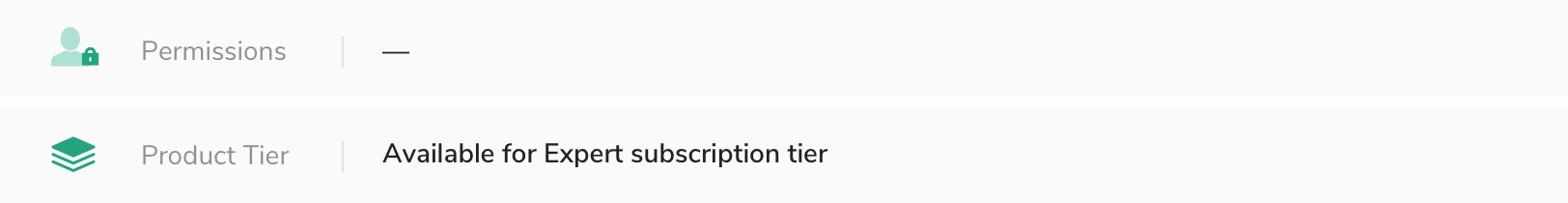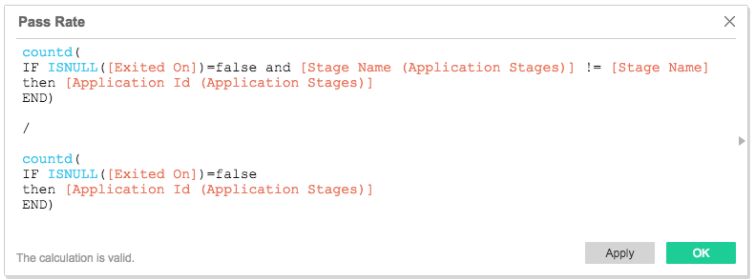# Calculate Pass Rates Based on Applicants That Enter/Exit a StageThe default Pass Rate measure in the BI Connector data set (and in the Pipeline report in Greenhouse) is an *implied* pass rate that assumes applicants have passed through all previous stages in order to get to their current stage, regardless of whether or not they were actually advanced through each of those stages in Greenhouse.

For example, if you add a candidate into Greenhouse directly into a Phone Interview stage, we assume that the candidate has passed every previous stage (e.g. Application Review) in order to make it to the Phone Interview stage, even if the candidate never technically entered or exited the Application Review stage in Greenhouse.

If no stages are skipped, this pass rate will be the exact same as if you were to calculate it by counting the number of candidates that completed a stage and moved on, divided by the total number of candidates that completed a stage. However, if any stages are skipped (e.g. application review, or an optional phone interview or take-home test), then the implied pass rate will be different than the pass rate calculated based on actual Greenhouse usage.

To calculate pass rates based on actual Greenhouse usage, you can apply the following methodology to data from the application_stages table (which represents every stage an application could be in, and the enter/exit times of that stage) and the applications table (which represents the current stage of an application).

Note: The example below uses Tableau.Text-formula you can copy-and-paste:

countd(
IF ISNULL([Exited On])=false and [Stage Name (Application Stages)] != [Stage Name]
then [Application Id (Application Stages)]
END)

/

countd(
IF ISNULL([Exited On])=false
then [Application Id (Application Stages)]
END)

Explanation of the formula:

• The denominator represents the total number of candidates that completed a stage. You can read it as "if there is a value for when an application exited the stage (i.e. the value is not null), then count the application"
• The numerator represents the total number of candidates that completed a stage and moved on. You'll note that the formula is the exact same as the denominator, except for the additional of a second logical condition that evaluates whether or not the stage that was completed is equal to the applicant's current stage. If they are equal, the applicant has completed the stage and been rejected. If they are different, the applicant has completed the stage and moved into another stage (Note: Here, we assume that applicants only ever move forward, and not backward).